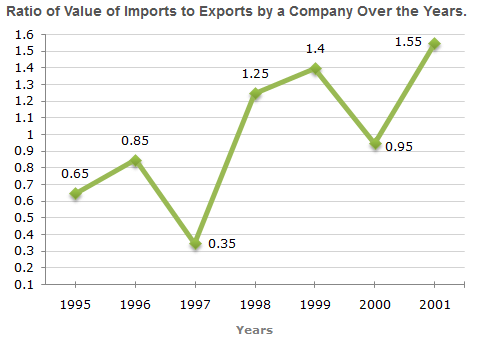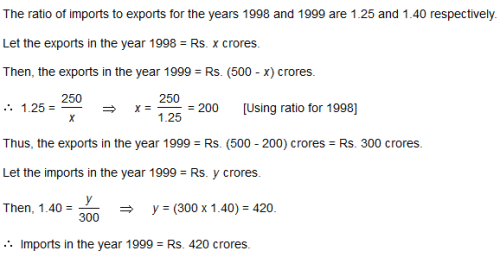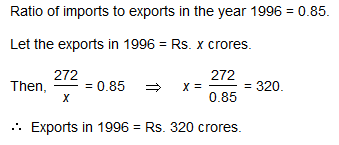# Test: Line Charts- 2

## 5 Questions MCQ Test IBPS Clerk Prelims - Study Material, Mock Tests | Test: Line Charts- 2

Description
Attempt Test: Line Charts- 2 | 5 questions in 10 minutes | Mock test for Banking Exams preparation | Free important questions MCQ to study IBPS Clerk Prelims - Study Material, Mock Tests for Banking Exams Exam | Download free PDF with solutions
QUESTION: 1

### Practice Quiz or MCQ (Multiple Choice Questions) with solutions are available for Practice, which would help you prepare for "Line Charts" under LR and DI. You can practice these practice quizzes as per your speed and improvise the topic. The same topic is covered under various competitive examinations like - CAT, GMAT, Bank PO, SSC and other competitive examinations. Q. The following line graph gives the ratio of the amounts of imports by a company to the amount of exports from that company over the period from 1995 to 2001.If the imports in 1998 was Rs. 250 crores and the total exports in the years 1998 and 1999 together was Rs. 500 crores, then the imports in 1999 was ?

Solution:QUESTION: 2

### The following line graph gives the ratio of the amounts of imports by a company to the amount of exports from that company over the period from 1995 to 2001.The imports were minimum proportionate to the exports of the company in the year ?

Solution:

The imports are minimum proportionate to the exports implies that the ratio of the value of imports to exports has the minimum value.

Now, this ratio has a minimum value 0.35 in 1997, i.e., the imports are minimum proportionate to the exports in 1997.

QUESTION: 3

### The following line graph gives the ratio of the amounts of imports by a company to the amount of exports from that company over the period from 1995 to 2001.What was the percentage increase in imports from 1997 to 1998 ?

Solution:

The graph gives only the ratio of imports to exports for different years. To find the percentage increase in imports from 1997 to 1998, we require more details such as the value of imports or exports during these years.

QUESTION: 4

The following line graph gives the ratio of the amounts of imports by a company to the amount of exports from that company over the period from 1995 to 2001.If the imports of the company in 1996 was Rs. 272 crores, the exports from the company in 1996 was ?

Solution:QUESTION: 5

The following line graph gives the ratio of the amounts of imports by a company to the amount of exports from that company over the period from 1995 to 2001.In how many of the given years were the exports more than the imports ?

Solution:

The exports are more than the imports imply that the ratio of value of imports to exports is less than 1.

Now, this ratio is less than 1 in years 1995, 1996, 1997 and 2000.

Thus, there are four such years.Use Code STAYHOME200 and get INR 200 additional OFF Use Coupon Code